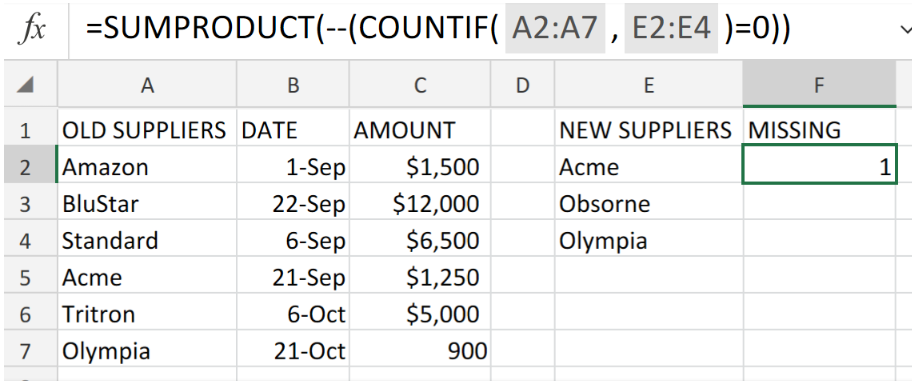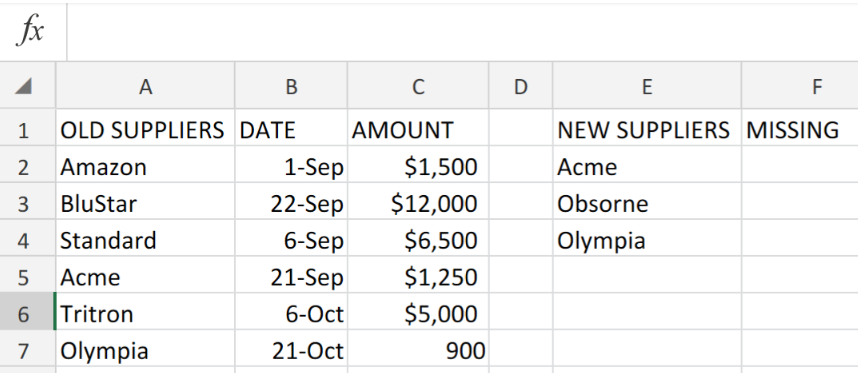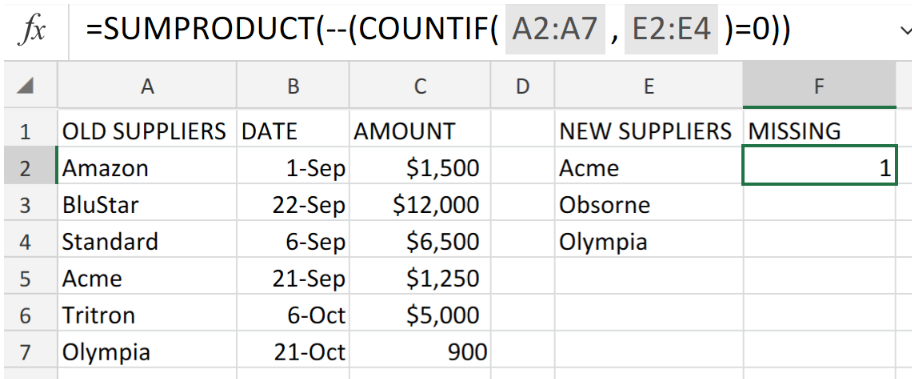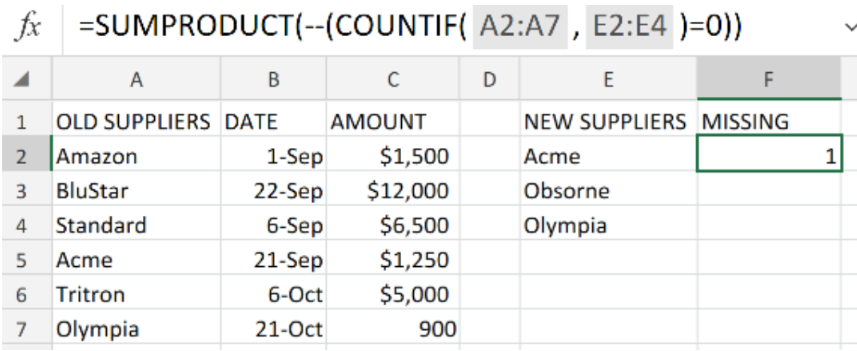Get instant live expert help with Excel or Google Sheets“My Excelchat expert helped me in less than 20 minutes, saving me what would have been 5 hours of work!”

#### Post your problem and you’ll get Expert help in seconds.

Your message must be at least 40 characters
Our professional Expert are available now. Your privacy is guaranteed.

# Count Missing Values in ExcelFigure 1. of Count Missing Values in Excel.

In the event that we are required to the data values available in a list, but happen to be missing/omitted from a separate list, we can utilize a formula syntax which is based on the following two Excel Functions – SUMPRODUCT and COUNTIF Functions. This tutorial will step through the process.

## Generic Formula

`=SUMPRODUCT(--(COUNTIF(list1,list2)=0))`

## How to Use the SUMPRODUCT and COUNTIF Functions in Excel.

The COUNTIF Function in Excel references data values in a range against specified criteria.

We will utilize the SUMPRODUCT Function in Excel to add up the data values in our range and determine any missing data values.

We can achieve this by following four simple steps.

1. Arrange our total set of data value which we want to check out for missing items, in one column.

In the example illustrated below, we have labeled the column as OLD SUPPLIERS.

Our purposes here is to check out the listed suppliers for a retail store against a fresh list of suppliers.Figure 2. of List of Data values in Excel.

1. Be sure to make provision for a column in our worksheet where Excel can return items found or not found. In the example illustrated above, we have labeled this column as MISSING.
2. Enter the following formula syntax into the formula bar for cell F2 of our worksheet;

`=SUMPRODUCT(--(COUNTIF(A2:A7,E2:E4)=0))`Figure 3. of Count Missing Values in Excel.

Excel returns 1 data value (Osborne) as missing from our list1.

1. Copy the formula syntax in cell F2 above and enter it into the remaining cells in column F (MISSING) to get our desired results.

## Note

Our total range (list1) of data values in the example illustrated above, is A2:A7

Our list of data values to be checked for missing items (list2) in the example illustrated above, is E2:E4Figure 4. of Final Result.

## Instant Connection to an Expert through our Excelchat Service:

Our live Excelchat Service is here for you. We have Excel Experts available 24/7 to answer any Excel questions you may have. Guaranteed connection within 30 seconds and a customized solution for you within 20 minutes.

### Did this post not answer your question? Get a solution from connecting with the expert.Another blog reader asked this question today on Excelchat:
Solution examplesi have a table with Dates, First names , Last names and i want to count how many times a name occurs, but if a name occurs more than once on a particular date i need to only count it once. this is on excel and not google sheets
Solved by F. H. in 31 minsI need a formula to count the number of rows that contain at least one of two possible phrases, but not give me the total number of instances that both phrases occur overall.
Solved by E. B. in 33 minsNeed a formula that counts (1,2,3,4 ect..in cell M21) a range of cells, N4:N20, which are annual premium values. But I only want to add a sale count if the average monthly premium is above \$30 per sale. So if someone has 4 sales but they don't equal a total of \$120/mth (\$30 x 4 = \$120)... they would only get a count of 3 sales.
Solved by S. E. in 60 minsHello, I am trying to create a total color column? Can anyone help?
Solved by I. A. in 60 minsAny rep that has orders over 500 and occurrences at zero for each month, for a 6 month period. I need a sum
Solved by C. E. in 60 mins## Subscribe to Excelchat.coAnother blog reader asked this question today on Excelchat: